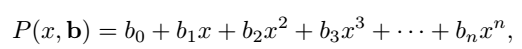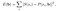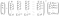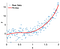# Linear regression with polynomials

In my previous two stories “Linear regression with straight lines” and “Linear regression with quadratic equations”, I explained how to find the optimum parameters of specific functions so as to minimize the sum squared error between a fit function y(x) and a data set S(x), using the method of least squares analysis (LSA). Now, I will extend these methods to a polynomial of any degree.

# Mathematical DerivationEquation 1: polynomial of degree n

since the numbered parameters b0, b1, and so on start at n = 0, we will require a total of n+1 equations in order to solve for all these coefficients. As before, we define the sum squared error function asEquation 2: sum-squared error function

and taking partial derivatives we end up with the general formula (after applying the chain rule for partial derivatives)

Now, equating the derivatives to zero, we end up with the system

and now we can writeEquation 3: solution to the parameters b

# Implementation in MATLAB

%%%%%%%%%%%%%%%%%%%%%%%%%%%%%%%%%%%%%%%

% Least-Squares-Analysis (LSA) for n-order polynomial fit

%

% Description: generates a random data-set around a polynomial curve with

% preset values and then uses LSA matrix methods to find the coefficients

% of the line of best-fit, and overlays the fit-line to the dataset:

% Equation → y = b0 + b1*x + b2*x² + b3*x³ + … + b{n}*x^{n}

%

% Made by: Oscar A. Nieves

% Made in: 2019

%%%%%%%%%%%%%%%%%%%%%%%%%%%%%%%%%%%%%%%

clear all; close all; clc;

%% Generate dataset

x = 0:0.01:2; % x-axis

% Set ‘guess’ for order of polynomial needed

N_guess = 6;

% Generate N random coefficients for polynomial using a Uniform Distribution

% between the values [a,b]

a = -1;

b = 1;

N = 4; % order of dataset polynomial

coeffs = a + (b — a)*rand(N+1,1);

coeffs_ran = a + (b — a)*rand(N+1,1);

% dataset

S = 0;

for k = 1:N+1

S = S + coeffs(k)*x.^(k-1) + coeffs_ran(k).*randn(size(x));

end

%% Polynomial LSA

n = N_guess;

for k = 1:2*n+1

row_A(k) = sum( x.^(k-1) );

end

for k = 1:n+1

A(k,:) = row_A(k:end-n+k-1);

RHS(k) = sum( S.*x.^(k-1) );

end

% convert b into a column vector

if size(RHS,1) == 1

RHS = RHS.’;

end

% Compute coefficient vector

b = A\RHS;

% Fit polynomial to data

y = 0;

for k = 1:n+1

y = y + b(k).*x.^(k-1);

end

% Calculate R² coefficient of determination

R2_quad = sum( (y — mean(S)).² )./sum( (S — mean(S)).² );

disp([‘R² = ‘ num2str(R2_quad)]);

% Plots results

figure(1);

set(gcf,’color’,’w’);

scatter(x,S); hold on;

plot(x,y,’r’,’LineWidth’,3); hold off;

legend(‘Raw Data’,’Fit-line’); legend boxoff;

legend(‘Location’,’northwest’);

xlabel(‘x’);

ylabel(‘y’);

axis tight;

set(gca,’FontSize’,20);

%%%%%%%%%%%%%%%%%%%%%%%%%%%%%%%%%%%%%%%

A single run of the code yields the plot in Figure 1.Figure 1: Polynomial fit (n=6) on a random polynomial data set (n=4). R² = 0.8675

Oscar is a physicist, educator and STEM enthusiast. He is currently finishing a PhD in Theoretical Physics with a focus on photonics and stochastic dynamics.

## More from Oscar Nieves

Oscar is a physicist, educator and STEM enthusiast. He is currently finishing a PhD in Theoretical Physics with a focus on photonics and stochastic dynamics.